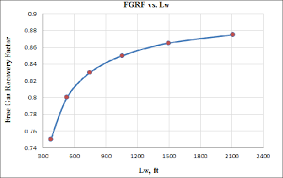## How to Calculate and Solve for Gas Recovery Factor of a Reservoir Fluid Flow | The Calculator EncyclopediaThe image above represents a gas recovery factor.

To compute for the gas recovery factor, three essential parameters are needed and these parameters are initial pressure(Pi), initial compressibility factor (Ziand P/Z Ratio (P/Z).

The formula for calculating the gas recovery factor:

RFG = 1 – P/Z.Pi / Zi

Where;

RFG = Gas Recovery Factor (P, Z)
Pi = Initial Pressure
Zi = Initial Compressibility Factor
P/Z = P/Z Ratio

Let’s solve an example;
Find the gas recovery factor when the initial pressure is 12, the initial compressibility factor is 7 and the P/Z ratio is 36.

This implies that;

Pi = Initial Pressure = 12
Zi = Initial Compressibility Factor = 7
P/Z = P/Z Ratio = 36

RFG = 1 – P / Z.Pi / Zi
RFG = 1 – 36[12 / 7]
RFG = 1 – 36[1.714]
RFG = 1 – 61.714
RFG = -60.714

Therefore, the gas recovery factor is -60.714.